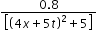NEET  >  Test: Behavior of Progressive Waves

# Test: Behavior of Progressive Waves

Test Description

## 10 Questions MCQ Test Physics Class 11 | Test: Behavior of Progressive Waves

Test: Behavior of Progressive Waves for NEET 2023 is part of Physics Class 11 preparation. The Test: Behavior of Progressive Waves questions and answers have been prepared according to the NEET exam syllabus.The Test: Behavior of Progressive Waves MCQs are made for NEET 2023 Exam. Find important definitions, questions, notes, meanings, examples, exercises, MCQs and online tests for Test: Behavior of Progressive Waves below.
Solutions of Test: Behavior of Progressive Waves questions in English are available as part of our Physics Class 11 for NEET & Test: Behavior of Progressive Waves solutions in Hindi for Physics Class 11 course. Download more important topics, notes, lectures and mock test series for NEET Exam by signing up for free. Attempt Test: Behavior of Progressive Waves | 10 questions in 10 minutes | Mock test for NEET preparation | Free important questions MCQ to study Physics Class 11 for NEET Exam | Download free PDF with solutions
 1 Crore+ students have signed up on EduRev. Have you?
Test: Behavior of Progressive Waves - Question 1

### As a wave propagates; which of the following is not satisfied?

Test: Behavior of Progressive Waves - Question 2

### The disc of a siren containing 60 holes rotates at a constant speed of 360 rpm. The emitted sound is in unison with a tuning fork of frequency.

Detailed Solution for Test: Behavior of Progressive Waves - Question 2

Frequency of revolution of disc = 360 rpm = 360 / 60rps = 60rps
Frequency of emitted sound = 6 × No.of holes
= 6 ×60 = 360Hz.

Test: Behavior of Progressive Waves - Question 3

### The speed of transverse waves in a string depends on:

Detailed Solution for Test: Behavior of Progressive Waves - Question 3

For a string v = √(T/μ)

Test: Behavior of Progressive Waves - Question 4

Speed of the sound in a perfectly rigid rod would be

Detailed Solution for Test: Behavior of Progressive Waves - Question 4

Since particles in a rigid body do not move and hence, no time is delayed in travelling of sound waves.

Test: Behavior of Progressive Waves - Question 5

Which instrument work on the basis of reflection of sound waves?

Detailed Solution for Test: Behavior of Progressive Waves - Question 5

Stethoscope works on the basis of reflection of sound waves.

Test: Behavior of Progressive Waves - Question 6

Speed of a progressive wave,v is given by

Detailed Solution for Test: Behavior of Progressive Waves - Question 6

f = v/λ
v = λf

Test: Behavior of Progressive Waves - Question 7

What causes the rolling sound of thunder?

Detailed Solution for Test: Behavior of Progressive Waves - Question 7

The air is not of uniform density as we go upwards. as we go upwards there is a gradual decrease in air density which results in refraction thereby leading to the rolling sound of thunder.

Test: Behavior of Progressive Waves - Question 8

Properties of a kind of wave are given below. Identify the type of wave

i. Wave can not be detected by human ear
ii. Velocity of wave in free space is 3x108m/s
iii. Velocity is not effected by temperature
iv. Waves can travel through vacuum

Detailed Solution for Test: Behavior of Progressive Waves - Question 8

These are the properties of radio waves. No possible explanation.

Test: Behavior of Progressive Waves - Question 9

Y (x,t) =Syntax error from line 1 column 49 to line 1 column 73. Unexpected ‘mathsize’. represents a moving pulse where X and y are n metres and t in second. Then which of the following dose not hold true?

Detailed Solution for Test: Behavior of Progressive Waves - Question 9

t = 0 & x = 0
y = 0.16 m
ymax = 0.16 m
Pulse is symmetric because y (x) = y (-x)
t = 1s
x=-1.25m
y is again 0.16m
Therefore speed is 1.25m/s
So it travels 2.5 m in 2 sec

Test: Behavior of Progressive Waves - Question 10

The speed of transverse waves on a string depends upon

Detailed Solution for Test: Behavior of Progressive Waves - Question 10

The speed of a wave on a string depends on the square root of the tension divided by the mass per length, the linear density.

## Physics Class 11

127 videos|464 docs|210 tests
 Use Code STAYHOME200 and get INR 200 additional OFF Use Coupon Code
Information about Test: Behavior of Progressive Waves Page
In this test you can find the Exam questions for Test: Behavior of Progressive Waves solved & explained in the simplest way possible. Besides giving Questions and answers for Test: Behavior of Progressive Waves, EduRev gives you an ample number of Online tests for practice

## Physics Class 11

127 videos|464 docs|210 tests

### How to Prepare for NEET

Read our guide to prepare for NEET which is created by Toppers & the best Teachers